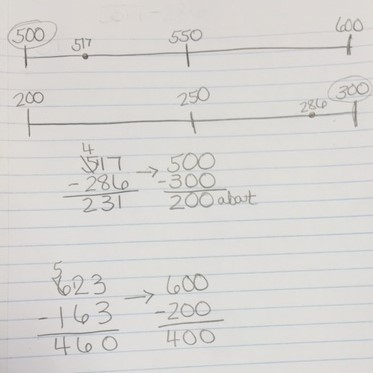## ArchivesEarlier this year, students built conceptual understanding of multiplication of whole numbers and decimals and multiplication of decimals by decimals. They used concrete models and grid paper to model the multiplication to find the product. The standard, MAFS.5.NBT.2.7, requires students…In 4th grade students also use rounding to estimate sums and differences. This strategy is used to make sure that their estimation is reasonable. They used this strategy in 3rd grade. Below is some examples of estimation that students learned…